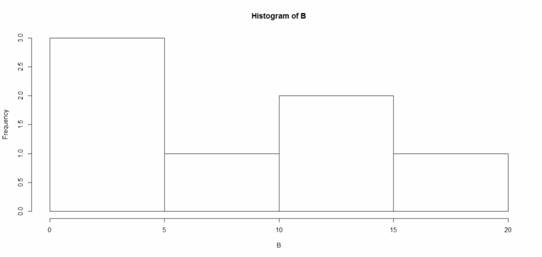# histogram

### Member Training: Preparing to Use (and Interpret) a Linear Regression Model

November 1st, 2020 byYou think a linear regression might be an appropriate statistical analysis for your data, but you’re not entirely sure. What should you check before running your model to find out?

### What R Commander Can do in R Without Coding–More Than You Would Think

October 19th, 2015 by

I received a question recently about R Commander, a free R package.R Commander overlays a menu-based interface to R, so just like SPSS or JMP, you can run analyses using menus.  Nice, huh?

The question was whether R Commander does everything R does, or just a small subset.

Unfortunately, R Commander can’t do everything R does. Not even close.

But it does a lot. More than just the basics.

So I thought I would show you some of the things R Commander can do entirely through menus–no programming required, just so you can see just how unbelievably useful it is.

Since R commander is a free R package, it can be installed easily through R! Just type `install.packages("Rcmdr")` in the command line the first time you use it, then type `library("Rcmdr")` each time you want to launch the menus.

### Data Sets and Variables

Import data sets from other software:

• SPSS
• Stata
• Excel
• Minitab
• Text
• SAS Xport
` `` `` `` `

Define Numerical Variables as categorical and label the values

Open the data sets that come with R packages

Merge Data Sets

Edit and show the data in a data spreadsheet

Personally, I think that if this was all R Commander did, it would be incredibly useful. These are the types of things I just cannot remember all the commands for, since I just don’t use R often enough.

### Data Analysis

Yes, R Commander does many of the simple statistical tests you’d expect:

• Chi-square tests
• Paired and Independent Samples t-tests
• Tests of Proportions
• Common nonparametrics, like Friedman, Wilcoxon, and Kruskal-Wallis tests
• One-way ANOVA and simple linear regression

What is surprising though, is how many higher-level statistics and models it runs:

• Hierarchical and K-Means Cluster analysis (with 7 linkage methods and 4 options of distance measures)
• Principal Components and Factor Analysis
• Linear Regression (with model selection, influence statistics, and multicollinearity diagnostic options, among others)
• Logistic regression for binary, ordinal, and multinomial responses
• Generalized linear models, including Gamma and Poisson models

In other words–you can use R Commander to run in R most of the analyses that most researchers need.

### Graphs

A sample of the types of graphs R Commander creates in R without you having to write any code:

• QQ Plots
• Scatter plots
• Histograms
• Box Plots
• Bar Charts

The nice part is that it does not only do simple versions of these plots.  You can, for example, add regression lines to a scatter plot or run histograms by a grouping factor.

### R is Not So Hard! A Tutorial, Part 12: Creating Histograms & Setting Bin Widths

March 7th, 2014 byI’m sure you’ve heard that R creates beautiful graphics.

It’s true, and it doesn’t have to be hard to do so.  Let’s start with a simple histogram using the hist() command, which is easy to use, but actually quite sophisticated.

First, we set up a vector of numbers and then we create a histogram.

`B <- c(2, 4, 5, 7, 12, 14, 16)`
`hist(B)`That was easy, but you need more from your histogram. (more…)